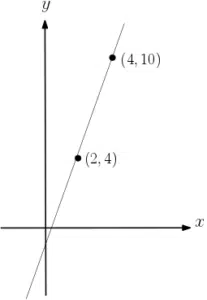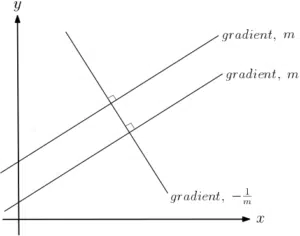# Straight Lines

You will be expected to be able to find the equation of a straight line given a variety of scenarios. This could be as simple as finding the equation of a line given two points, or it could be finding the equation of a parallel or perpendicular line given points or other relevant information. You may also be expected to represent straight lines in different formats including $y=mx+c$, $ax+by+c=0$, or $y-y_1=m(x-x_1)$. Recall that the equation of a straight line is $y=mx+c$ where $m$ is the gradient and $c$ is the $y$-intercept. Take the Coordinate Geometry course.

## Line Equations given Two PointsRecall that to find the equation of a straight line between two points, you must first find the gradient of the line that connects the two points. We can calculate this gradient using rise over run. One of the points can then be used to calculate the y-intercept of the line.

## Parallel and PerpendicularYou may be required to find the equation of a straight line that is either parallel or perpendicular to another given line. This could be directly where you asked to find an equation in no uncertain terms. Alternatively, the question may be stated indirectly, where you are asked for the area of a triangle, for example, which requires finding the equations first. Note that parallel lines have the same gradient.
If the gradient of a given line is $m$ then the gradient of the line perpendicular to it is $-\frac{1}{m}$.Dr Nat

### AS Mechanics SUVAT Equations

asmaths
5 Lectures
0 HoursDr Nat

### AS Maths Vectors

asmaths
15 Lectures
0 HoursDr Nat

### AS Maths Coordinate Geometry

asmaths
15 Lectures
0 HoursDr Nat

### AS Maths Binomial Expansion

asmaths
11 Lectures
0 HoursDr Nat

asmaths
17 Lectures
0 Hours